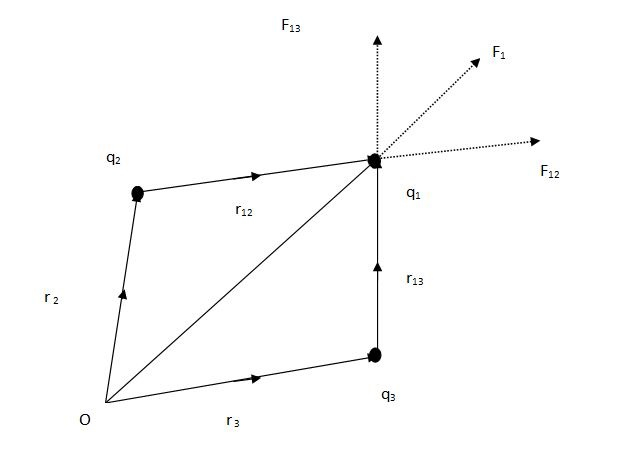# Superposition Principle and Continuous Charge Distribution

## Introduction

Electric charges in the field of electricity and of magnetism, display the fundamental property of particles of matter. Both positive and negative charges exist together within a circuit and therefore display their different characters.

For example, when the accumulation of one kind of charge exceeds then it results in positing the character of repealing, or when both the charges present in exceeding amount then they show the charges are attracted to each other. Electrons are known to be the negative charges and protons are known as positive charges.

## Electric Charge and its Properties

Electric charge can be referred to as the physical property for any matter that intends to get an exertion of force or pressure when these matters are placed within a field of magnetism. Electromagnetism is a phenomenon that shows the interaction that occurs within the fields of electricity and of magnetic fields (Anastopoulos & Hu, 2020).

However, a charge can be measured with the help of natural units that can be referred to as equivalent to the proton or electron charges and are considered to be the fundamental physical constant.

## Conductors and Insulators

Conductors are defined as the substances that support in conducting of electricity through them; on the other hand, insulators are substances that do allow the passing of electricity through them. In conducting materials, electrons flow freely from one end to another and the distribution of charge is noticed all over the body and including, for example, water, bodies of humans and metals (Doskoch & Man’ko, 2019).

On the other hand, the movement of electrons is less or negligible and seems to remain at a fixed place and its examples include glass, plastic and wood.

## Coulomb’s Law

Coulomb’s Law defines the notion of force that is noticed between any two specified point charges. However, it states that the force that is exerted between these two specified charges is inversely proportional (Old.amu.ac, 2022).

The force is said to be directly proportional to the magnitudes associated with these charges and this force acts on the line joining both the points.

## Definition of the Superposition Principle

Principle of Superposition is defined as the net current that is observed within a part of the linear circuits. This net current is said to be equal to the sum of currents expressed in an algebraic form that is separately generated by each of the sources.

It can be expressed as,

$\mathrm{F_{net}=F_{12}+F_{13}=(1/4*\pi\varepsilon_{0})/[(q_{1}*q_{2})/r_{{12}^{2}}*r_{12}+(q_{1}*q_{2})/r_{{13}^{2}}*r_{13}]}$respectively## What is known as the Continuous Charge Distribution?

The term continuous charge distribution is defined as the charges that are bound in closed vicinity and with a relatively negligible presence of space between the charges. The notion of charge density is applicable for displaying how charges can get accumulated within a specified point. However, there are three types noticed for the continuous distribution of charges.

Images Coming soon

## Linear Charge Distribution

In the distribution of linear charges, the charges seem to get evenly distributed along all through the length of the object. For example, it can be a straight wire and the charges that are distributed are represented by the symbol, $\mathrm{\lambda}$ and are measured with the unit of coulombs per meter.

Images Coming soon

## Surface charge distribution

In the surface charge distribution, is said to be, when the charges are uniformly distributed over a specified area, for example, such as that of a circular disk. This is represented by the symbol, σ and is measured by coulombs per square meter.

Images Coming soon

## Volume Charge Distribution

Volume charge distribution is stated when electric charges are evenly distributed over a particular volume, for example, the distribution of charges that are seen in spheres or in cylinder structures. However, this is measured with the help of coulombs per cubic meter and is denoted by $\mathrm{\rho}$ respectively.

Images Coming soon

## Conclusion

In this tutorial, the focus has been given to determining what are electric changes and how they are related to the phenomenon of superimposition that is noticed in the field of electrostatic. More to this it is noted that the distribution of electric charges is dependent on the nature of kinds of continuous charge distribution.

## FAQs

Q.1. What are the limitations acknowledged in Coulomb’s law?

Ans. There are many advantages noticed in coulomb’s law that are as follows, the first one of which is coulomb’s law is not applicable for expanded masses, only the charges that are stationary in nature allow coulomb’s law. In addition to these, the distances between nuclear distributions are not guaranteed if they increase or decrease for the stated limit of 10 to 15 meters. It is also stated that this law is not applicable for the computation of minor charges.

Q.2. What is the meaning of point distribution of charges?

Ans. The term point distribution of charges signifies when a charge is distributed to a specific. However, the distribution of mass should be considered with respect to the notion of area, distance travelled and volume. This kind of distribution of charges is rarely made use of, whereas, the distributions of linear charge, surface charges and volume charges depend upon the shape of the object and its dimensions.

Q.3. What are the disadvantages and advantages associated with the application of the theorem of superposition?

Ans. The advantages of the theorem of superposition include its applicability in both the nodes and its respective sources, massively used for the analysis of circuits and also asked for the transformation of any kind of circuits into the equivalent of Norton or Thevenin. On the other, this theory does not imply the notion of power.